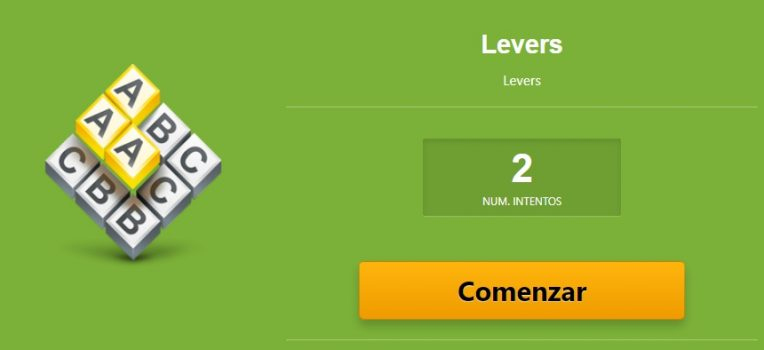# Levers

## Linear transmission of motion

Linear transmission mechanisms transmit motion and force through a motor to another point.

# Levers

A lever is a rigid bar that turns around a point called a fulcrum. At one end of the bar, force (F) is applied to move a resistance or load (R) at the other end of the bar.

We say the lever is in equilibrium when the forces acting on opposite ends of a lever are equal, that is: the result of the force (F) multiplied by the distance (d) from the fulcrum is the same as the result of the load (R) multiplied by the distance (r) from the fulcrum.

We can express this mathematically as the Law of the Lever

F x d = R x r

There are three types of levers 🙋

Class 1Class 2Class 3

The fulcrum is between the force and the resistance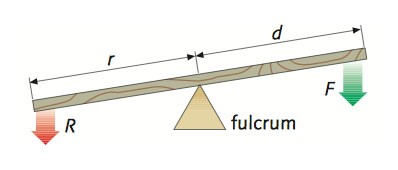The effect of the force applied is increased or decreased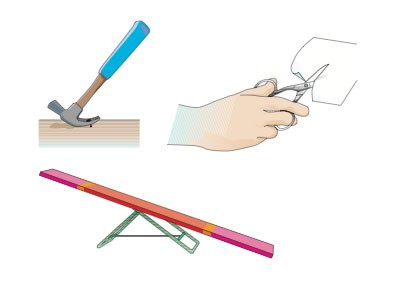The resistance is between the fulcrum and the force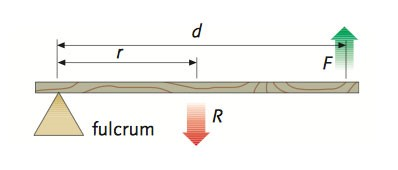The effect of the force applied is always increased ( d > r )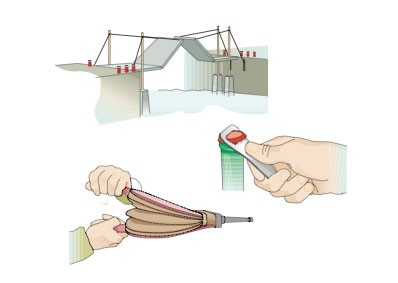The force is between the fulcrum and the resistance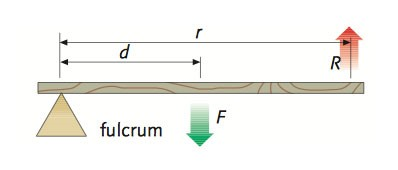The effect of the force applied is always decreased ( d < r )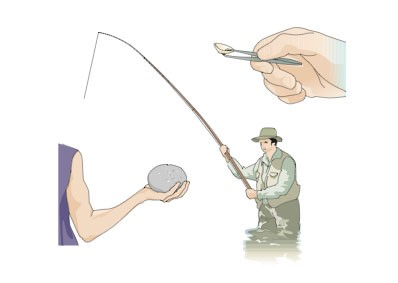Exercise 1

Exercise:  Draw in your notebook each of the following levers. Indicate, for each case, in which direction the balance is inclined: towards the right, left or in equilibrium: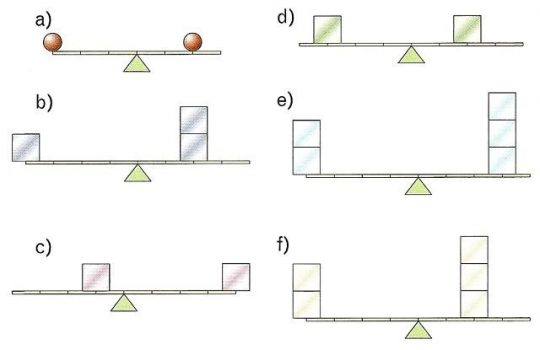Exercise 2

Exercise:  Draw the crane of the picture in your notebook . The crane seen below needs to lift a pallet of cement sacks which weight 5.000N. The counterweight is 10.000N and is located at the 5m mark on the tower. At what distance of the tower will the pallet be lifted so the structure does not suffer?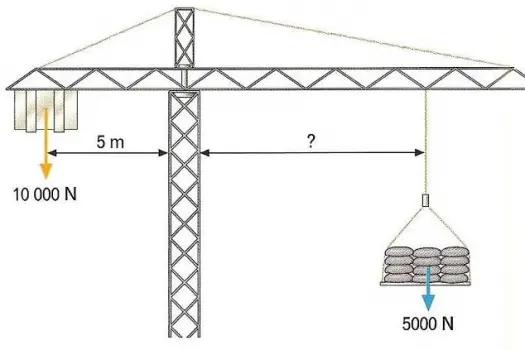Exercise 3

Exercise:  Draw the wheelbarrow of the picture in your notebook. If have a force of 500N, what weight am I able to transpor with the wheelbarrow drawn below?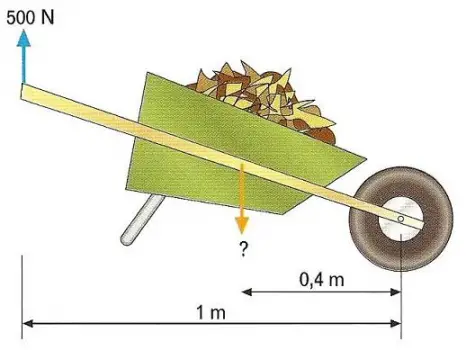Online Test

In the following activity you must choose different levers per group depending on its type.